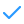Synonyms:

# annual hours, yearly hours

What is Annualized Hours

Annualized hours are the total number of hours worked in a year, divided by the number of weeks in a year.

Annualized Hours explained

Annualized hours are a way of calculating the number of hours an employee works over the course of a year. This method of calculation takes into account the number of hours worked in a week, the number of weeks worked in a year, and the number of hours worked in a year. This method of calculation is often used by employers to calculate the number of hours an employee works in a year so that they can properly compensate the employee.

Find and engage
1 billion candidates

One search engine to find and reach talent across the entire web1 billion profilesContact infoFree